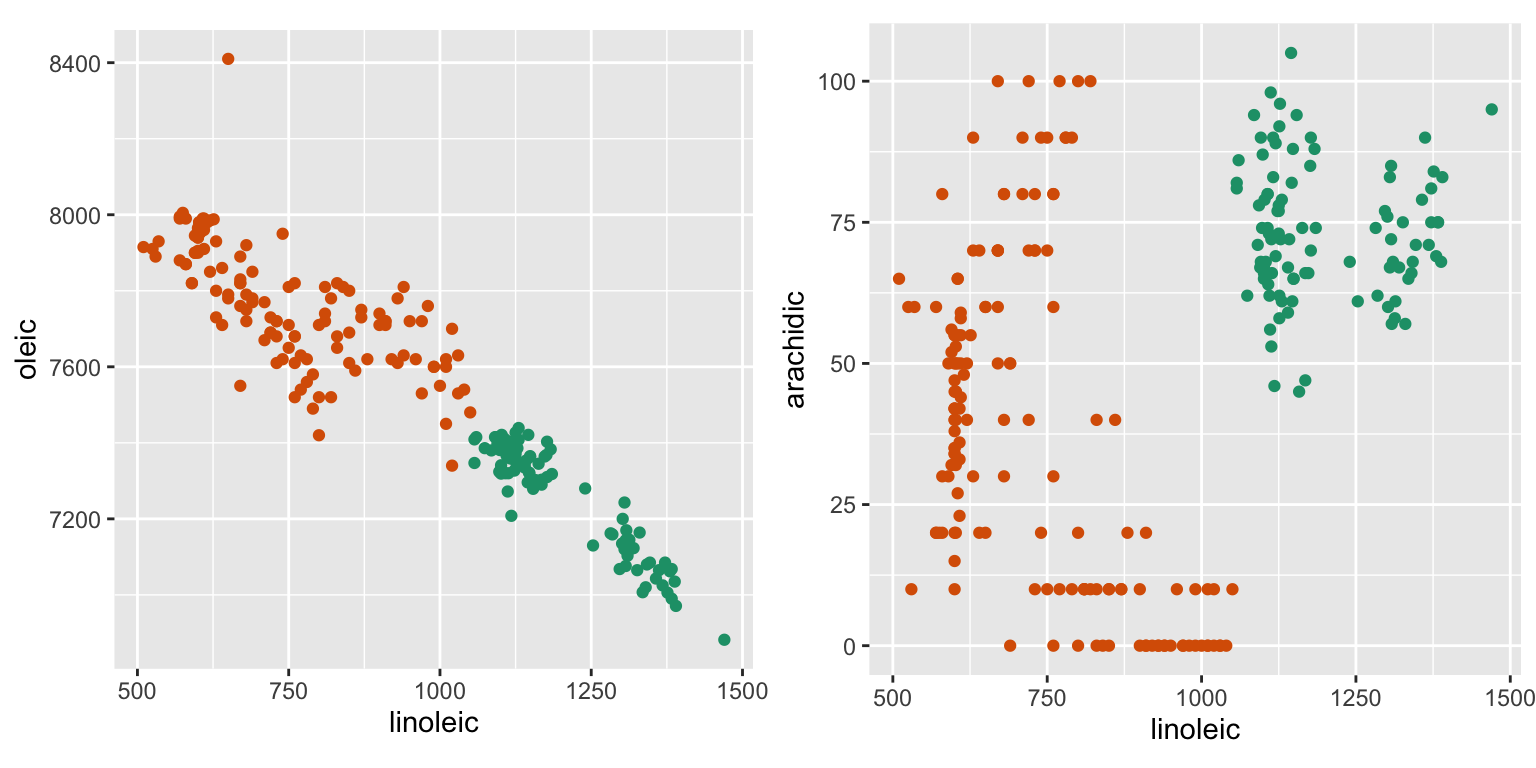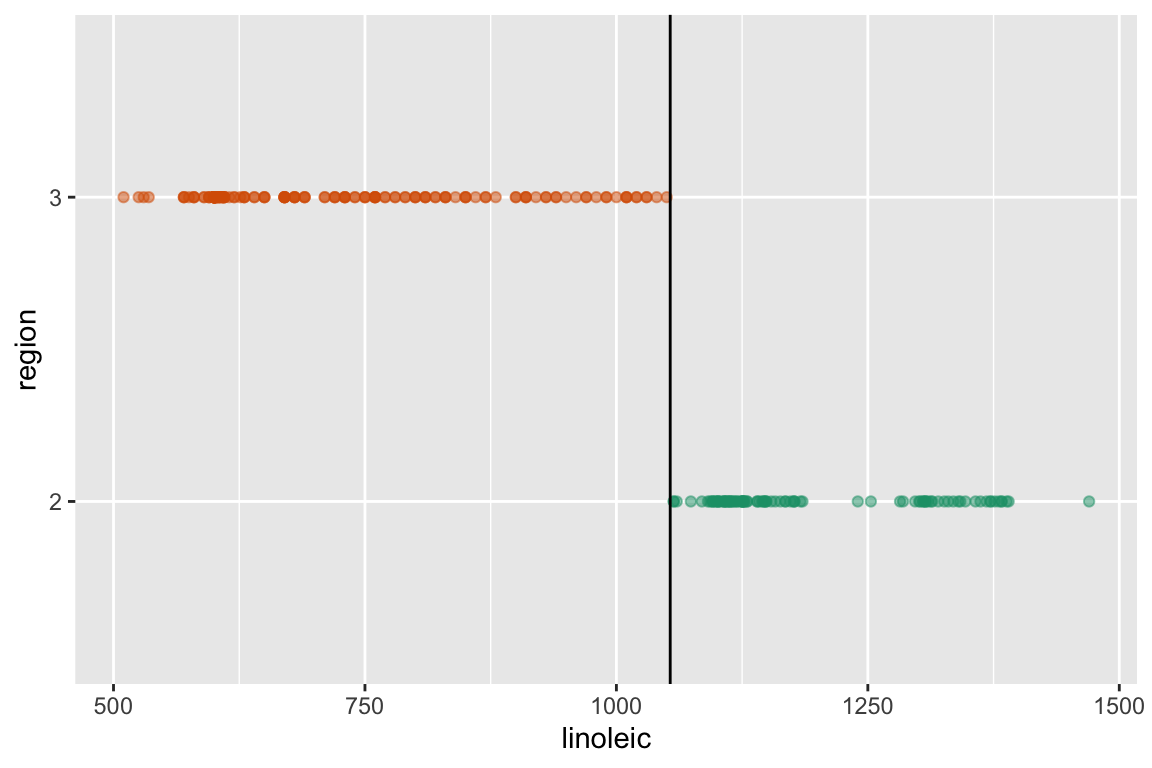# Objective

The objectives for this week are:

• Practice fitting a classification tree model
• Understand the way the tree model is fitted based on impurity
• Learn about the relationship between fitting parameters, bias and variance

# Class discussion

We will focus on two of the three main groups, north, sardinia, in the olive oil data, and think about how to best separate the two groups using the eight variables.

region palmitic palmitoleic stearic oleic linoleic linolenic arachidic eicosenoic
2 1129 120 222 7272 1112 43 98 2
2 1042 135 210 7376 1116 35 90 3
2 1103 96 210 7380 1085 32 94 3
2 1118 97 221 7279 1154 35 94 2
2 1052 95 215 7388 1126 31 92 1

Question 1: Here are plots of two pairs of variables. If you got to choose two variables for splitting the two groups, which would you choose, oleic or arachidic, in association with linoleic? Why?Question 2: For the olive oil data set, the classification tree will use just one of the possible eight variables for its model. It splits on linoleic acid as shown. Why do you think the tree fitting algorithm chose this variable? There is no gap between the groups. What problem might this create with future data? Why?

# n= 249
#
# node), split, n, loss, yval, (yprob)
#       * denotes terminal node
#
# 1) root 249 98 3 (0.3935743 0.6064257)
#   2) linoleic>=1053.5 98  0 2 (1.0000000 0.0000000) *
#   3) linoleic< 1053.5 151  0 3 (0.0000000 1.0000000) *Question 3: Suppose you work with linoleic and arachidic. Would quadratic discriminant analysis produce a better separation than the tree? Argue your viewpoint.

Question 4: Find a linear combination of linoleic and arachidic, and create a new variable to pass to the tree. Re-fit the tree with this variable instead of the original two. What does the model look like now? Is this better than the original tree?

Question 5: In general, why is it often important to create new variables (feature engineering) when building models?

# Practice

1. This question is about entropy as an impurity metric for a classification tree.

1. Write down the formula for entropy as an impurity measure for two groups.

2. Establish that the the worst case split has 50% one group and 50% the other group, in whatever way you would like (algebraicly or graphically).

3. Extend the entropy formula so that it can be used to describe the impurity for a possible split of the data into two subsets. That is, it needs to be the sum of the impurity for both left and right subsets of data.

2. For this sample of data,

1. compute the entropy impurity metric for all possible splits.

2. Write down the classification rule for the tree that would be formed for the best split.

3. For the following data set, compute. Write out the decision tree, and also sketch the boundary between classes.

1. olive oils, for three regions

2. chocolates, for type

3. flea, for species

4. For the crabs data, make a new variable combining species and gender into one class variable.

1. Use the grand and guided tour with the LDA index to examine the data. Describe the shape. Between LDA and a classification tree which d you expect to perform better on this data?

2. Fit the default decision tree, using species and gender. Explain why it is so complicated.

3. Break the data into 50% training data, and 50% test data, ensuring that sampling is done within the class variable. Change the options for the tree to fit it the training data with increasingly well. Compute the training and test error for each of the options and plot these. What best options would be suggested based on minimising test error?